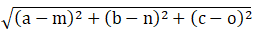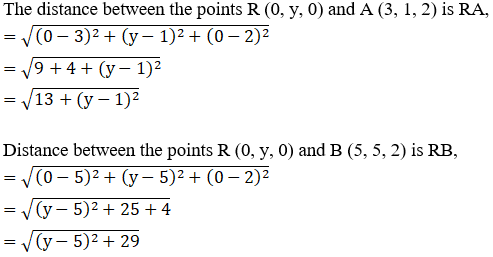Find the point on y-axis which is equidistant from the points (3, 1, 2) and (5, 5, 2).

Asked by Sakshi | 1 year ago |  30

##### Solution :-

Given:

The points (3, 1, 2) and (5, 5, 2)

We know x = 0 and z = 0 on y-axis

Let R(0, y, 0) any point on the y-axis

According to the question:

RA = RB

RA2 = RB2

By using the formula,

The distance between any two points (a, b, c) and (m, n, o) is given by,So,We know, RA2 = RB2

13+ (y – 1)2 = (y – 5)2 + 29

y2+ 1 – 2y + 13

= y2+ 25 – 10y + 29

10y – 2y = 54 – 14

8y = 40

y = $$\dfrac{40}{8}$$

= 5

The point R (0, 5, 0) on y-axis is equidistant from (3, 1, 2) and (5, 5, 2).

Answered by Aaryan | 1 year ago

### Related Questions

#### A(1, 2, 3), B(0, 4, 1), C(-1, -1, -3) are the vertices of a triangle ABC. Find the point in which the bisector of the

A(1, 2, 3), B(0, 4, 1), C(-1, -1, -3) are the vertices of a triangle ABC. Find the point in which the bisector of the angle ∠BAC meets BC.

#### The mid-points of the sides of a triangle ABC are given by (-2, 3, 5), (4, -1, 7) and (6, 5, 3). Find the coordinates

The mid-points of the sides of a triangle ABC are given by (-2, 3, 5), (4, -1, 7) and (6, 5, 3). Find the coordinates of A, B and C.

#### If the points A(3, 2, -4), B(9, 8, -10) and C(5, 4, -6) are collinear, find the ratio in which C divided AB.

If the points A(3, 2, -4), B(9, 8, -10) and C(5, 4, -6) are collinear, find the ratio in which C divided AB.